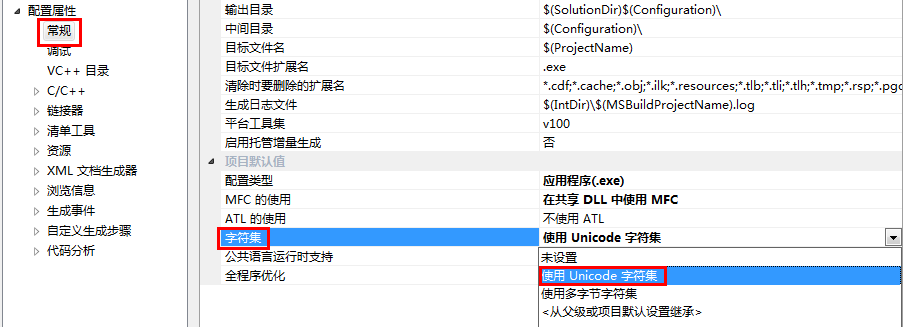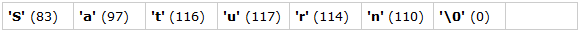关于Unicode编码

2012/04/23

简述ANSI与Unicode

``````char cResponse; // 'Y' or 'N'
// str* functions 和
wchar_t cResponse; // 'Y' or 'N'
// wcs* functions 替换成：
#include<TCHAR.H> // Implicit or explicit include
TCHAR cResponse; // 'Y' or 'N'
// _tcs* functions
````````````#ifdef _UNICODE
typedef wchar_t TCHAR;
#else
typedef char TCHAR;
#endif
``````

`_UNICODE`宏的作用就是当项目”设置为Unicode字符集“时, `TCHAR`含义是`wchar_t`；”设置为多字节字符集“时, `TCHAR`含义是`char`.

strlen的原型如下：

``````size_t strlen(const char*);
``````

wcslen的原型如下：

``````size_t wcslen(const wchar_t* );
``````

``````size_t _tcslen(const TCHAR* );
``````

wc表示宽字符, 可知`wcs`表示宽字符串, 同理可知`_tcs`表示_T字符串, 能猜到_T在逻辑上既代表`char`又代表`wchar_t`了吧?

``````#ifdef _UNICODE
#define _tcslen wcslen
#else
#define _tcslen strlen
#endif
``````

`TCHAR.H`中, 会找到与之相似的准确定义.

``````void _TPrintChar(char);
``````

``````void _TPrintChar(wchar_t);
``````

``````void PrintCharA(char); // A = ANSI
void PrintCharW(wchar_t); // W = Wide character
``````

``````#ifdef _UNICODE
void _TPrintChar(wchar_t);
#else
void _TPrintChar(char);
#endif
``````

``````TCHAR cChar;
_TPrintChar(cChar);
``````

``````// WinUser.H
#ifdef UNICODE
#define SetWindowText  SetWindowTextW
#else
#define SetWindowText  SetWindowTextA
#endif // !UNICODE
``````

ANSI与Unicode的转换

``````"This is ANSI String. Each letter takes 1 byte"
``````

``````L"This is Unicode string. Each letter would take 2 bytes, including spaces."
``````

``````"ANSI String"; // ANSI
L"Unicode String"; // Unicode

_T("Either string, depending on compilation"); // ANSI or Unicode
// or use TEXT macro, if you need more readability
``````

``````// SIMPLIFIED
#ifdef _UNICODE
#define _T(c) L##c
#define TEXT(c) L##c
#else
#define _T(c) c
#define TEXT(c) c
#endif
``````

`##`符号是符号连接操作符, 它将`_T("Unicode")`替换为`L"Unicode"`, 该替换取决于宏参数——`_UNICODE`是否被定义. 如果没有被定义, `_T("Unicode")`意味着`"Unicode"`. 符号连接操作符并不仅仅是VC或字符编码中的特定符号, 它甚至存在于C语言中.

``````char c = 'C';
char str = "MyProject";

_T(c);
_T(str);
``````

``````error C2065: 'Lc' : undeclared identifier
error C2065: 'Lstr' : undeclared identifier
``````

``````BOOL SetWindowText(HWND, const TCHAR*);
``````

``````BOOL SetWindowTextA(HWND, const char*);
BOOL SetWindowTextW(HWND, const wchar_t*);
``````

``````HMODULE hDLLHandle;
FARPROC pFuncPtr;
//pFuncPtr will be null, since there doesn't exist any function with name SetWindowText !
``````

`SetWindowTextA``SetWindowTextW`都由`User32.dll`所导出的. 并没有通用的函数名.

``````[DllImport("user32.dll")]
extern public static int SetWindowText(IntPtr hWnd, string lpString);
``````

ANSI与Unicode互转的操作并不局限于GUI函数, 而涵盖了整个Windows API系列中拥有两套方案的字符串处理函数. 例如：

• `CreateProcess`
• `GetUserName`
• `OpenDesktop`
• `DeleteFile`
• etc

更好的指针表示

`TCHAR`往往修饰单个字符, 当然可以声明一个`TCHAR`的数组. 如果你想表示一个character-pointer或一个const-character-pointer, 会用下列哪一个呢?

``````// ANSI characters
foo_ansi(char*);
foo_ansi(const char*);
/*const*/ char* pString;

// Unicode/wide-string
foo_uni(WCHAR*);
wchar_t* foo_uni(const WCHAR*);
/*const*/ WCHAR* pString;

// Independent
foo_char(TCHAR*);
foo_char(const TCHAR*);
/*const*/ TCHAR* pString;
``````

``````size_t strlen(const char*);
``````

``````size_t strlen(LPCSTR);
``````

`LPCSTR`符号的定义类型是：

``````// Simplified
typedef const char* LPCSTR;
``````

• LP – Long Pointer
• C – Constant
• STR – String

`LPCSTR`的本质含义是指向固定字符串的(长)指针.

``````LPSTR strcpy(LPSTR szTarget, LPCSTR szSource);
``````

szTarget的类型是`LPSTR`,少了C, 定义如下：

``````typedef char* LPSTR;
``````

``````size_t nLength;
nLength = wcslen(L"Unicode");
``````

`wcslen`函数原型：

``````size_t wcslen(const wchar_t* szString); // Or WCHAR*
``````

``````size_t wcslen(LPCWSTR szString);
``````

``````typedef const WCHAR* LPCWSTR;
// const wchar_t*
``````

• LP - Pointer
• C - Constant
• WSTR - Wide character String

``````wchar_t* wcscpy(wchar_t* szTarget, const wchar_t* szSource)
``````

``````LPWSTR wcscpy(LPWSTR szTarget, LPCWSTR szSource);
``````

``````size_t _tcslen(const TCHAR* szString); 或
size_t _tcslen(LPCTSTR szString);
``````

• LP - Pointer
• C - Constant
• T = TCHAR
• STR = String

``````size_t _tcscpy(TCHAR* pTarget, const TCHAR* pSource); 或, 更加通用的形式：
size_t _tcscpy(LPTSTR pTarget, LPCTSTR pSource);
``````

用法举例：

``````int main()
{
TCHAR name[] = "Saturn";
int nLen; // Or size_t

lLen = strlen(name);
}
``````

``````error C2440: 'initializing' : cannot convert from 'const char ' to 'TCHAR []'
error C2664: 'strlen' : cannot convert parameter 1 from 'TCHAR []' to 'const char *'
``````

``````TCHAR name[] = (TCHAR*)"Saturn";
``````

``````nLen = wcslen("Saturn");
// ERROR: cannot convert parameter 1 from 'const char ' to 'const wchar_t *'
``````

``````nLen = wcslen((const wchar_t*)"Saturn");
``````

`"Saturn"`是一个7字节的顺序序列：``````TCHAR name[] = _T("Saturn");
``````

``````wcslen(L"Saturn");
``````

``````lLen = strlen ((const char*)name);
``````

Unicode字符串是可以包含非英文字符的, 那样的结果就是无法预测的了.

字符数与字节数

``````BOOL SetCurrentDirectory( LPCTSTR lpPathName );
DWORD GetCurrentDirectory(DWORD nBufferLength,LPTSTR lpBuffer);
``````

``````TCHAR sCurrentDir;

// Pass 255 and not 255*2
GetCurrentDirectory(sCurrentDir, 255);
``````

``````LPTSTR pBuffer; // TCHAR*

pBuffer = new TCHAR; // Allocates 128 or 256 BYTES, depending on compilation.
``````

``````pBuffer = (TCHAR*) malloc (128 * sizeof(TCHAR) );
``````